# The Milky Way GalaxyPage 4

#### WATCH ALL SLIDES

Bracket values from Francis and Anderson (2009)

Local Standard of Rest

Slide 18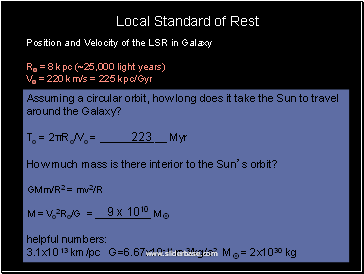## Position and Velocity of the LSR in Galaxy

Ro = 8 kpc (~25,000 light years)

Vo = 220 km/s = 225 kpc/Gyr

Assuming a circular orbit, how long does it take the Sun to travel around the Galaxy?

To = 2πRo/Vo = _ Myr

How much mass is there interior to the Sun’s orbit?

GMm/R2 = mv2/R

M = Vo2Ro/G = M

3.1x1013 km/pc G=6.67x10-11 m3/kg/s2 M = 2x1030 kg

Local Standard of Rest

223

9 x 1010

Slide 19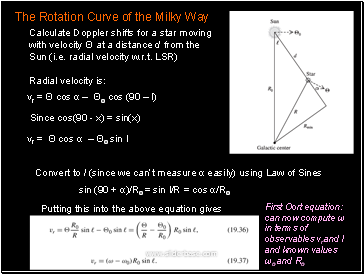Calculate Doppler shifts for a star moving with velocity Θ at a distance d from the Sun (i.e. radial velocity w.r.t. LSR)

Since cos(90 - x) = sin(x)

Convert to l (since we can’t measure α easily) using Law of Sines

Putting this into the above equation gives

The Rotation Curve of the Milky Way

vr = Θ cos α – Θo cos (90 – l)

vr = Θ cos α – Θo sin l

sin (90 + α)/Ro = sin l/R = cos α/Ro

First Oort equation: can now compute ω in terms of observables vr and l and known values ωo and Ro

Slide 20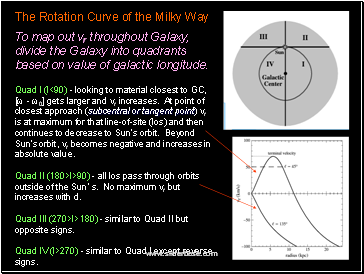To map out vr throughout Galaxy, divide the Galaxy into quadrants based on value of galactic longitude.

Quad I (l<90) - looking to material closest to GC, [ω - ω0] gets larger and vr increases. At point of closest approach (subcentral or tangent point) vr is at maximum for that line-of-site (los) and then continues to decrease to Sun’s orbit. Beyond Sun’s orbit, vr becomes negative and increases in absolute value.

Quad II (180>l>90) - all los pass through orbits outside of the Sun’s. No maximum vr but increases with d.

The Rotation Curve of the Milky Way

Slide 21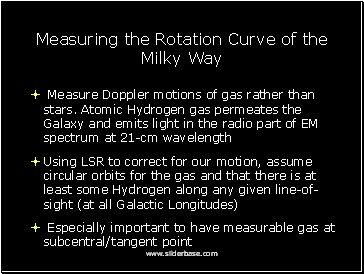## Measuring the Rotation Curve of the Milky Way

Measure Doppler motions of gas rather than stars. Atomic Hydrogen gas permeates the Galaxy and emits light in the radio part of EM spectrum at 21-cm wavelength

Using LSR to correct for our motion, assume circular orbits for the gas and that there is at least some Hydrogen along any given line-of-sight (at all Galactic Longitudes)

Especially important to have measurable gas at subcentral/tangent point

Slide 22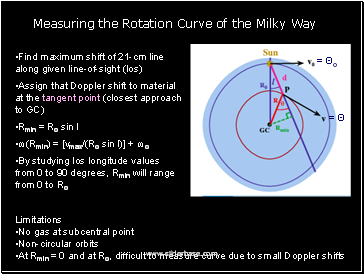Find maximum shift of 21-cm line along given line-of-sight (los)

Assign that Doppler shift to material at the tangent point (closest approach to GC)

Go to page:
1  2  3  4  5  6  7  8